# Sum every nth column in Excel

In this article, we will learn to return SUM price by weekdays in Excel.

In simple words, while working with price filled data. Sometimes, we need to find the sum of value having a condition. Condition is to get the sum of price values in separated columns.

For this article we will be needing the use the following functions:

Now we will make a formula out of these functions. Here we will be given a list of values and we need to get the sum of values lays in each multiple of given nth column.
Use the formula:

=SUMPRODUCT ( -- ( MOD ( COLUMN ( list ) - COLUMN ( first ) + 1 , n ) = 0 ) , list )

list : list of values along the column

first : first cell of the list which returns the column number

n : nth column values to add up.

Example:

All of these might be confusing to understand. So, let's test this formula via running it on the example shown below.

Here we have given data containing numbers. Here we need to find the SUM of numbers in each multiple of the 3rd column. So for that we have assigned lists in a column for the formula.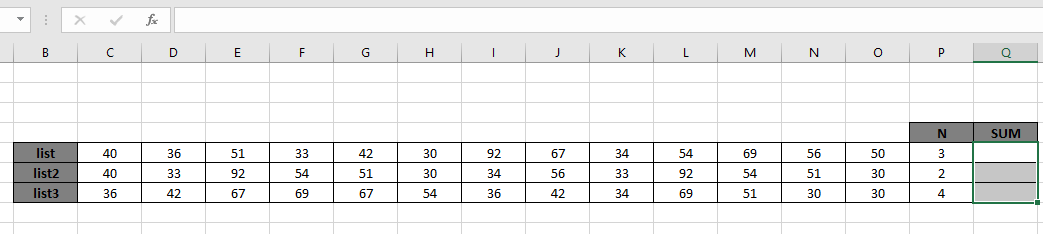Now we will use the below formula to get the SUM of numbers at 3rd, 6th, 9th, 12th and so on till the list ends
Formula:

=SUMPRODUCT ( -- ( MOD ( COLUMN ( list ) - COLUMN ( C5 ) + 1 , P5 ) = 0 ) ,list )

list : list of values along the column given as using the named ranges.

C5 : first cell of the list which returns the column number given as cell reference

P5 : value of n given as cell reference

Explanation:

• MOD(COLUMN(list)-COLUMN(C5)+1,P5) returns the relative column number for each n interval. Like

{ 1 , 2 , 0 , 1 , 2 , 0 , 1 , 2 , 0 , 1 , 2 , 0 , 1 }

• The above array will be matched with a 0 value and returns an array of True & False.

{ FALSE , FALSE , TRUE , FALSE , FALSE , TRUE , FALSE , FALSE , TRUE , FALSE , FALSE , TRUE , FALSE }

• SUMPRODUCT function considers value 1 for every TRUE value received and 0 for FALSE value as shown below.

=SUMPRODUCT ( -- ( { 0 , 0 , 1 , 0 , 0 , 1 , 0 , 0 , 1 , 0 , 0 , 1 , 0 } ),{ 40 , 36 , 51 , 33 , 42 , 30 , 92 , 67 , 34 , 54 , 69 , 56 , 50 } )

• -- converts TRUE to 1 and FALSE to 0.
• Values corresponding to 1s will get add up only as shown in the above explained formula.
• Now the SUMPRODUCT function adds up all the values as shown below.

=SUMPRODUCT ( { 0 , 0 , 51 , 0 , 0 , 30 , 0 , 0 , 34 , 0 , 0 , 56 , 0 } )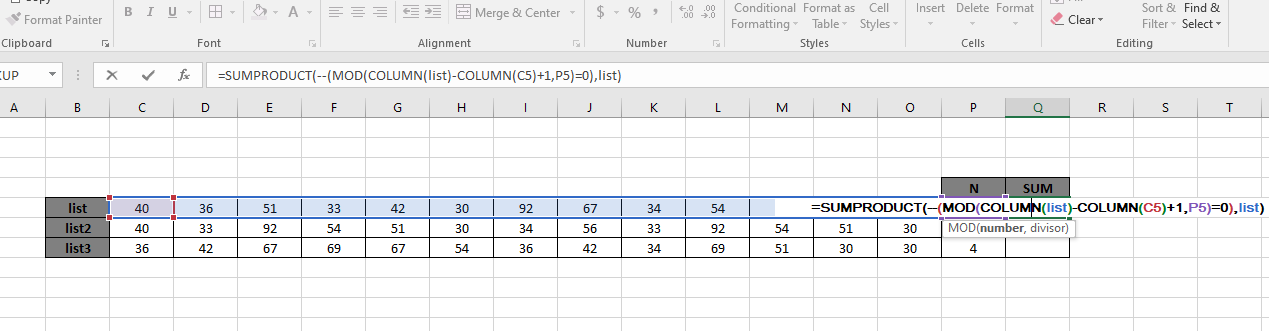The SUM of the values { 51 + 30 + 34 + 56 } must return 171.
Here the array to the function is given as named range and cell as cell_reference. Press Enter to get the result.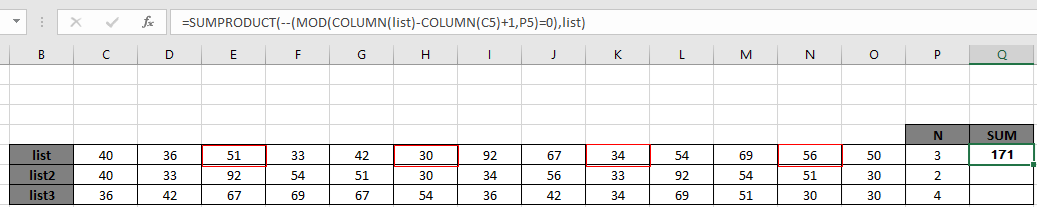As you can see in the above snapshot the sum of values extracted at each 3rd interval returns 171. The values are marked under red boxes as shown in the above snapshot.

Or you can use the list array using the below formula:

=SUMPRODUCT ( -- ( MOD ( COLUMN ( C5:O5 ) - COLUMN ( C5 ) + 1 , P5 ) = 0 ), C5:O5 )

The above formula will return the same result. There is only one replacement list named range is replaced with the array range (C5:O5).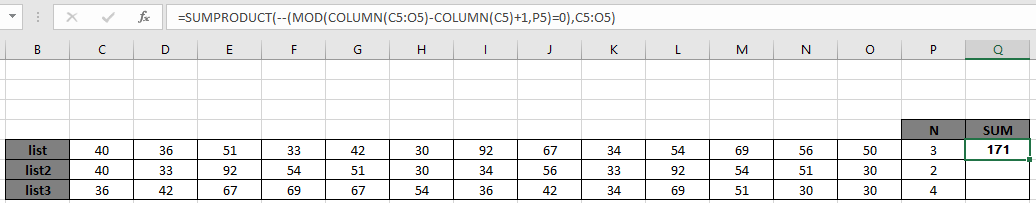As you can see the formula works fine. Now we need to copy the formula for the other lists. So use the CTRL + D or drag down option of excel.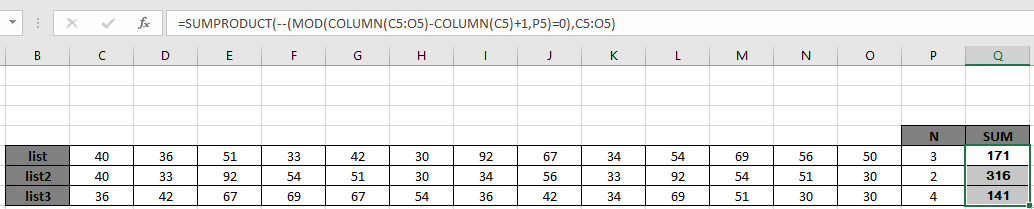As you can see from the above formula the you can get conditional values.

Notes:

1. The SUMPRODUCT function considers non - numeric values as 0s.
2. The SUMPRODUCT function considers logic value TRUE as 1 and False as 0.
3. The argument array must be of the same length, otherwise the formula returns error.

Hope this article about how to Return SUM only every nth column with formulas in Excel is explanatory. Find more articles on SUMPRODUCT functions here. Please share your query below in the comment box. We will assist you.

Related Articles

How to use the SUMPRODUCT function in Excel: Returns the SUM after multiplication of values in multiple arrays in excel.

SUM if date is between : Returns the SUM of values between given dates or period in excel.

Sum if date is greater than given date: Returns the SUM of values after the given date or period in excel.

2 Ways to Sum by Month in Excel: Returns the SUM of values within a given specific month in excel.

How to Sum Multiple Columns with Condition: Returns the SUM of values across multiple columns having condition in excel

Popular Articles

50 Excel Shortcut to Increase Your Productivity

Edit a dropdown list

Absolute reference in Excel

If with conditional formatting

If with wildcards

Vlookup by date

Convert Inches To Feet and Inches in Excel 2016

Join first and last name in excel

Count cells which match either A or B

Terms and Conditions of use

The applications/code on this site are distributed as is and without warranties or liability. In no event shall the owner of the copyrights, or the authors of the applications/code be liable for any loss of profit, any problems or any damage resulting from the use or evaluation of the applications/code.